If you need some time, we're serializing our recreational mathematics ebook, 24 Whimsical Clocks.  Here's chapter 2. Just click the blue image captions to try out the apps, and you can download them to put on your personal webpage.
 24 Whimsical Clocks: 2.  The Abacus Clock
This digital clock is made from an abacus with two columns for hours, two for minutes and two for seconds.  Like your hands, an abacus lets you count in fives and tens. The left pair of columns are for hours, the middle pair for minutes and the right pair for seconds. The bead at the top of each column counts for 5 single beads. The beads in the main compartment of the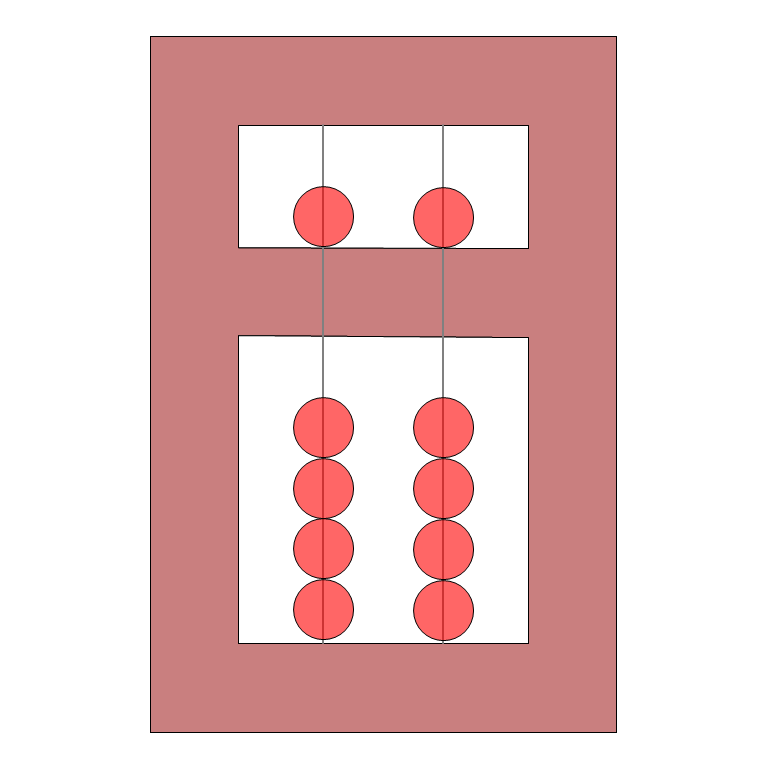Fig. 1.1 Abacus practice app
left column each count for 10 single beads. The bead in the top compartment, left column, counts as 50.  Figure 1.1 shows you how numbers between 0 and 99 are represented on two wires of the abacus.

Abacus arithmetic is still a competition sport in some countries (with a similar anachronistic quality that spelling competitions have in the United States).  You have to see a square root
extracted by an abacus champion to appreciate the power of this simple calculating tool in the right hands.

Here are a couple more examples.  See if you understand the time, then download the clockonto your desktop.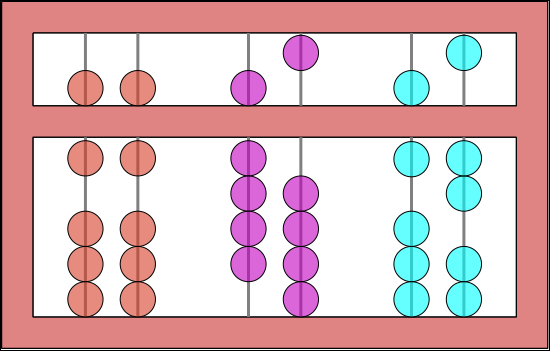Time 11:45:17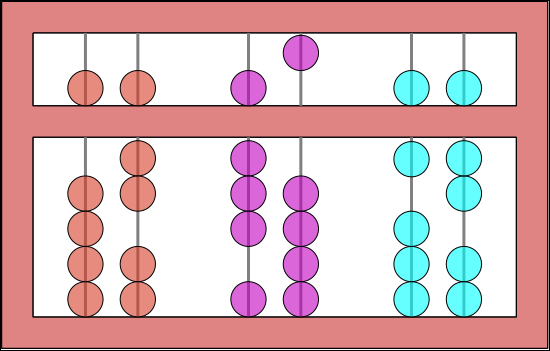Time 2:35:12October 2016Whimsical zero.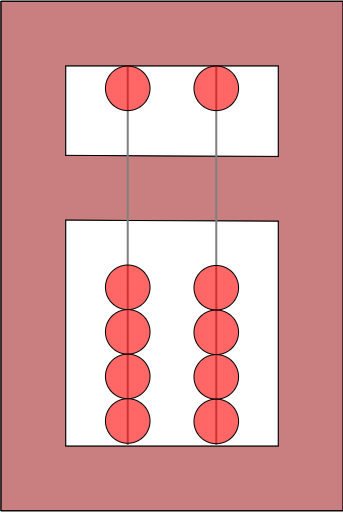Correct zero
Several readers pointed out that last month's whimsical clock uses an abacus in a quite unorthodox (some might say whimsical) way.

Our clock zeros the abacus by pulling all the beads vertically downwards. We are reliably informed, however, that standard usage of an abacus zeros away from the center line.

A corrected version of the clock is here.

This clock and 23 others may be found in our eBook
24 Whimsical Clocks.available on the iPad.

How to...create a whimsical clock in Geometry Expressions

When you export a JavaScript / HTML5 app from Geometry Expressions, there are a number of different forms that input variables can take. These include a text edit control, slider, advance button. You can also attach the variable to a timer.

If you attach a variable to a timer, the app will be presented with this variable's value animated. The variable will change between its lower and upper limits as set in the Variables Panel. Depending on what animation mode is set in the variables panel, it will either increase from its lower limit to its upper limit, then jump back to start again at the lower limit, or it will increase to the upper limit then decrease to the lower limit in a rocking back and forth motion.

How long it will take to complete its motion depends on what Timer Style you use.• Animation period - the time taken will be the number of seconds set in the animation duration entry in the Variables Panel.
• 1 minute period - the motion will be completed in 1 minute (can be used for the second hand of a whimsical clock).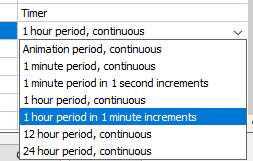• 1 hour period - the motion will be completed in 1 hour (can be used for the minute hand of a whimsical clock).
• 12 hour period - the motion will be completed in 12 hours (can be used for the hour hand of a 12-hour whimsical clock) .
• 24 hour period - the motion will be completed in 24 hours (useful for 24-hour whimsical clock).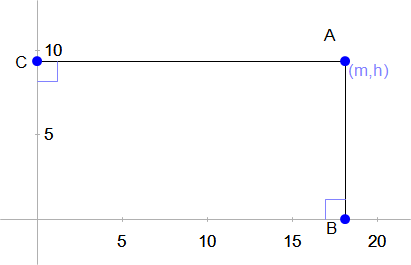We'll illustrate this by creating a whimsical clock consisting of a point on the Cartesian plane where you can read off the hour from its y coordinate and the minute from its x coordinate. You can see the resulting clock here.
• First turn on the axes, and make sure the labels are shown. Select the axes, then right click Axis Properties/Show Labels/Yes.
• Now draw a point and constrain its coordinates to be (m,h).
• Draw lines from the point to the axes and constrain these lines to be perpendicular to the axes.
• In the Variables Panel set the limits of variable m to be 0 and 60.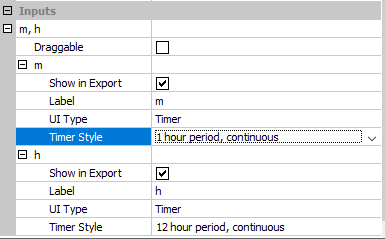• In the Variables Panel set the limits of variable h to be 0 and 12.
• Use File/Export/HTML5 JavaScript App.
• Make sure Auto Scale is not checked.
• In the Inputs section, uncheck Draggable on the input m,h.
• For variable m, check Show in Export, set UI type timer and Timer style 1 hour period continuous.
• For variable h, check Show in Export, set UI type timer and Timer style 12 hour period continuous.

Now when you browse to the exported html page, the point's coordinates should reflect the current time on your computer.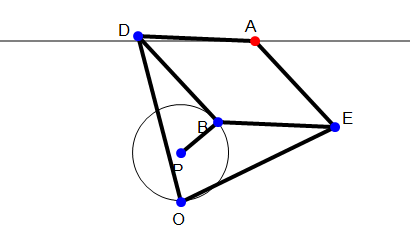Problem of the Month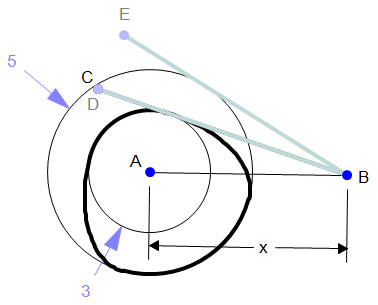A double-dwell cam is shaped from the arc of a circle radius 3cm and the arc of a concentric circle radius 5cm, along with smooth transition curves.

The cam rotates about the center of the circles A.

A flat face follower BC pivots around B.

The follower maintains tangential contact with the cam,

BD is the lower position of the follower (tangential to the inner circle).

BE is the higher position of the follower (tangential to the outer circle).

If the angle DBE is 12 degrees, what is the distance AB?

What is the largest angle DBC that can be achieved with this cam, if we change the distance between A and B?

Why Wait?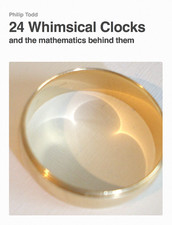If you enjoyed this clock with it's accompanying mathematics and you're eager for more, you don't have to wait for the next volume.  You can get the whole set here in a handy iBook!

Geometry Expressions does much more than just make Clock apps.
Get 10% off on your next copy -- use this coupon:  GX-45K2D47FDJ

A solution to September's ProblemThe diagram shows Paucellier's Straight Line Linkage.

Points O and P are fixed and

|PB|=|OP|=r

|OE|=|OD|=b

An app which lets you explore this ishere.

As B traverses a circle, point A traces a straight line. What is the perpendicular distance between P and the line traced by A?

How long is the line segment which can be traced by A?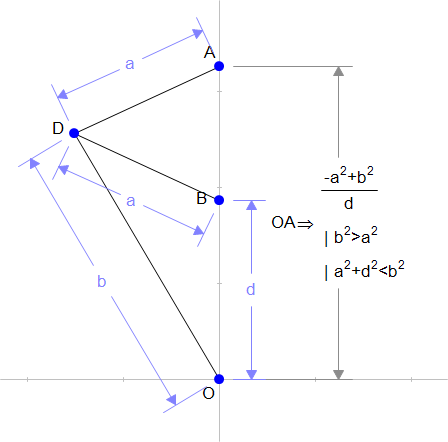We can consider the situation when A is vertically above O. At this point the result can be derived from applications of the Pythagorean theorem. Or Geometry Expressions can be used as illustrated.

At the furthest extent of the mechanism, we can see that OEA will be a straight line. If X is the position of point A at the extremity, then |OX| = a+b. The distance from here to the center line can be obtained by Pythagoras, or Geometry Expressions (see illustration). The length of segment traceable by A is double this quantity.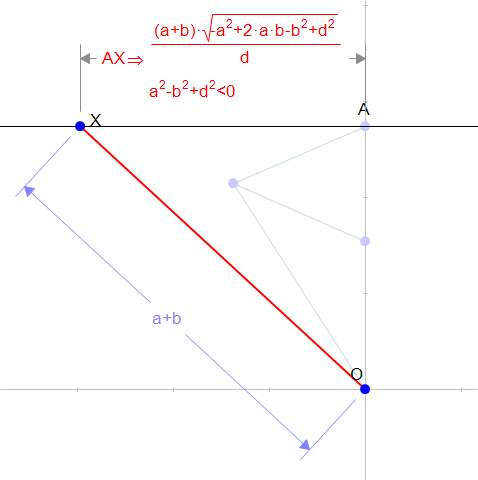info@paviathintegratedsolution.com www.paviathintegratedsolution.com